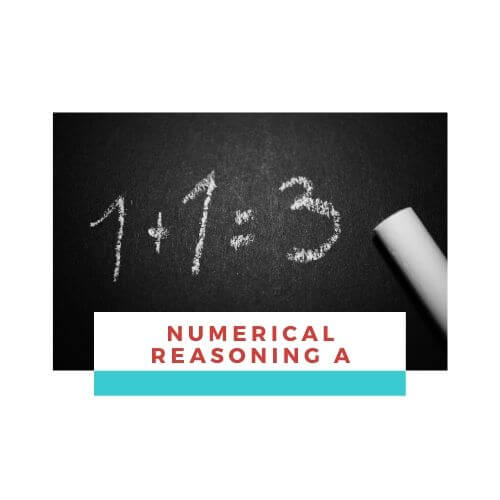## Numerical Reasoning A

##### Are you ready to take the upcoming Civil Service Exam?

Total items: 10 items
Duration: 10 minutes

Important: Prepare a pen and paper for math-related questions.

##### Topics
• Multiples and Factors
• Divisibility Rule
• Number Sequences
• PEMDAS
• Decimals
• Fractions
• Percent
• Ratio and Proportion
• Averages
• Word Problems

[sociallocker]

0%

You have 10 minutes to answer this quiz.Numerical Reasoning A

1 / 10

Car A is travelling at 67 kph while Car B is travelling at 59 kph. Both cars left the same place at the same time but in opposite directions. In how many hours will the two cars be 1,701 kilometers apart?

2 / 10

Using “Divisibility Rules”, which of the following is not divisible by 4?

3 / 10

The sum of a and b is 162, while c is equal to 39. What is the average of a, b and c?

4 / 10

2/18+17/45+7/12= ?

5 / 10

12.6 + 0.98 + 2.34 =

6 / 10

Find the 5th term in the sequence given by Xn = 3 (1/3)

7 / 10

20% of 125 is 40% of x. Find the value of x.

8 / 10

A pair of shoes is on sale at P2500, which is 80% its normal price. How much does the pair of shoes originally cost?

9 / 10

What is the value of 15(32 – 5 + 2 x 6) – 4 x 2  ?

10 / 10

The Greatest Common Factor (GCF) of 24 and 54 is:

0%

Did you like this quiz?

[/sociallocker]

## Explore Other Quizzes

#### Need More Quizzes?

Done answering FREE quizzes? Avail up to 50% OFF.

Z

#### Free Quizzes

Study and practice now using our FREE Quizzes.